SBOS458H December   2008  – June 2015

PRODUCTION DATA.

1. Features
2. Applications
3. Description
4. Revision History
5. Device Comparison Table
6. Pin Configuration and Functions
7. Specifications
8. Detailed Description
9. Application and Implementation
1. 9.1 Application Information
2. 9.2 Typical Applications
1. 9.2.1 Audio ADC Driver Performance: THS4521 and PCM4204 Combined Performance
3. 9.2.3 Differential Input to Differential Output Amplifier
4. 9.2.4 Single-Ended Input to Differential Output Amplifier
10. 10Power Supply Recommendations
11. 11Layout
12. 12Device and Documentation Support
13. 13Mechanical, Packaging, and Orderable Information

• D|8
• DGK|8
• DGK|8

## 7 Specifications

### 7.1 Absolute Maximum Ratings

Over operating free-air temperature range (unless otherwise noted).(1)
MIN MAX UNIT
Supply voltage, VS– to VS+ 5.5 V
Input/output voltage, VI (VIN±, VOUT±, VOCM pins) (VS–) – 0.7 (VS+) + 0.7 V
Differential input voltage, VID 1 V
Output current, IO 100 mA
Input current, II (VIN±, VOCM pins) 10 mA
Continuous power dissipation See Thermal Information table
Maximum junction temperature, TJ 150 °C
Maximum junction temperature, TJ (continuous operation, long-term reliability) 125 °C
Operating free-air temperature, TA –40 85 °C
Storage temperature, Tstg –65 150 °C
(1) Stresses beyond those listed under Absolute Maximum Ratings may cause permanent damage to the device. These are stress ratings only, which do not imply functional operation of the device at these or any other conditions beyond those indicated under Recommended Operating Conditions. Exposure to absolute-maximum-rated conditions for extended periods may affect device reliability.

### 7.2 ESD Ratings

VALUE UNIT
V(ESD) Electrostatic discharge Human body model (HBM), per ANSI/ESDA/JEDEC JS-001, all pins(1) ±1300 V
Charged device model (CDM), per JEDEC specification JESD22-C101, all pins(2) ±1000
Machine model (MM) ±50
(1) JEDEC document JEP155 states that 500-V HBM allows safe manufacturing with a standard ESD control process.
(2) JEDEC document JEP157 states that 250-V CDM allows safe manufacturing with a standard ESD control process.

### 7.3 Recommended Operating Conditions

over operating free-air temperature range (unless otherwise noted)
MIN NOM MAX UNIT
VS+ single-supply voltage 2.7 5.0 5.4 V
TA Ambient temperature –40 25 85 °C

### 7.4 Thermal Information

THERMAL METRIC(1) THS4521 THS4522 THS4524 UNIT
D DGK PW DBT
8 PINS 8 PINS 16 PINS 38 PINS
RθJA Junction-to-ambient thermal resistance 127.8 193.8 124.2 106.2 °C/W
RθJC(top) Junction-to-case (top) thermal resistance 81.8 84.1 62.8 60.9
RθJB Junction-to-board thermal resistance 68.3 115.3 68.5 65.5
ψJT Junction-to-top characterization parameter 32.2 17.9 15.8 18.5
ψJB Junction-to-board characterization parameter 67.8 113.6 68 65.1
RθJC(bot) Junction-to-case (bottom) thermal resistance N/A N/A N/A N/A

### 7.5 Electrical Characteristics: VS+ – VS– = 3.3 V

At VS+ = 3.3 V, VS– = 0 V, VOCM = open, VOUT = 2 VPP (differential), RL = 1 kΩ differential, G = 1 V/V, single-ended input, differential output, and input and output referenced to midsupply, unless otherwise noted.
PARAMETER TEST CONDITIONS TEST LEVEL(1) MIN TYP MAX UNIT
AC PERFORMANCE
Small-signal bandwidth VOUT = 100 mVPP, G = 1 C 135 MHz
VOUT = 100 mVPP, G = 2 C 49 MHz
VOUT = 100 mVPP, G = 5 C 18.6 MHz
VOUT = 100 mVPP, G = 10 C 9.3 MHz
Gain bandwidth product VOUT = 100 mVPP, G = 10 C 93 MHz
Large-signal bandwidth VOUT = 2 VPP, G = 1 C 95 MHz
Bandwidth for 0.1-dB flatness VOUT = 2 VPP, G = 1 C 20 MHz
Rising slew rate (differential) VOUT = 2-V Step, G = 1, RL = 200 Ω C 420 V/μs
Falling slew rate (differential) VOUT = 2-V Step, G = 1, RL = 200 Ω C 460 V/μs
Overshoot VOUT = 2-V Step, G = 1, RL = 200 Ω C 1.2%
Undershoot VOUT = 2-V Step, G = 1, RL = 200 Ω C 2.1%
Rise time VOUT = 2-V Step, G = 1, RL = 200 Ω C 4 ns
Fall time VOUT = 2-V Step, G = 1, RL = 200 Ω C 3.5 ns
Settling time to 1% VOUT = 2-V Step, G = 1, RL = 200 Ω C 13 ns
HARMONIC DISTORTION
2nd harmonic f = 1 MHz, VOUT = 2 VPP, G = 1 C –85 dBc
f = 1 kHz, VOUT = 1 VRMS, G = 1(3),
differential input
C –133 dBc
3rd harmonic f = 1 MHz, VOUT = 2 VPP, G = 1 C –90 dBc
f = 1 kHz, VOUT = 1 VRMS, G = 1(3),
differential input
C –141 dBc
Second-order intermodulation distortion Two-tone, f1 = 2 MHz, f2 = 2.2 MHz,
VOUT = 2-VPP envelope
C –83 dBc
Third-order intermodulation distortion Two-tone, f1 = 2 MHz, f2 = 2.2 MHz,
VOUT = 2-VPP envelope
C –90 dBc
Input voltage noise f > 10 kHz C 4.6 nV/√Hz
Input current noise f > 100 kHz C 0.6 pA/√Hz
Overdrive recovery time Overdrive = ±0.5 V C 80 ns
Output balance error VOUT = 100 mV, f ≤ 2 MHz (differential input) C –57 dB
Closed-loop output impedance f = 1 MHz (differential) C 0.3 Ω
Channel-to-channel crosstalk (THS4522, THS4524) f = 10 kHz, measured differentially C –125 dB
DC PERFORMANCE
Open-loop voltage gain (AOL) A 100 116 dB
Input-referred offset voltage TA = +25°C A ±0.2 ±2 mV
TA = –40°C to +85°C B ±0.5 ±3.5 mV
Input offset voltage drift(2) TA = –40°C to +85°C C ±2 μV/°C
Input bias current(4) TA = +25°C B 0.65 0.85 μA
TA = –40°C to +85°C B 0.75 0.95 μA
Input bias current drift(2) TA = –40°C to +85°C B ±1.75 ±2 nA/°C
Input offset current TA = +25°C B ±30 ±180 nA
TA = –40°C to +85°C B ±30 ±215 nA
Input offset current drift(2) TA = –40°C to +85°C B ±100 ±600 pA/°C
INPUT
Common-mode input voltage low TA = +25°C A –0.2 –0.1 V
TA = –40°C to +85°C B –0.1 0 V
Common-mode input voltage high TA = +25°C A 1.9 2 V
TA = –40°C to +85°C B 1.8 1.9 V
Common-mode rejection ratio (CMRR) A 80 100 dB
Input impedance C 0.7 pF kΩ∥pF
OUTPUT
Output voltage low TA = +25°C A 0.08 0.15 V
TA = –40°C to +85°C B 0.09 0.2 V
Output voltage high TA = +25°C A 3.0 3.1 V
TA = –40°C to +85°C B 2.95 3.05 V
Output current drive (for linear operation) RL = 50 Ω C ±35 mA
POWER SUPPLY
Specified operating voltage B 2.5 3.3 5.5 V
Quiescent operating current, per channel TA = +25°C A 0.9 1.0 1.2 mA
TA = –40°C to +85°C B 0.85 1.0 1.25 mA
Power-supply rejection ratio (±PSRR) A 80 100 dB
POWER DOWN
Enable voltage threshold Assured on above 2.1 V A 1.6 2.1 V
Disable voltage threshold Assured off below 0.7 V A 0.7 1.6 V
Disable pin bias current C 1 μA
Power-down quiescent current C 10 μA
Turn-on time delay Time to VOUT = 90% of final value, VIN= 2 V, RL = 200 Ω B 108 ns
Turn-off time delay Time to VOUT = 10% of original value, VIN= 2 V, RL = 200 Ω B 88 ns
VOCM VOLTAGE CONTROL
Small-signal bandwidth C 23 MHz
Slew rate C 55 V/μs
Gain A 0.98 0.99 1.02 V/V
Common-mode offset voltage from VOCM input Measured at VOUT with VOCM input driven, VOCM = 1.65 V ±0.5 V B ±2.5 ±4 mV
Input bias current VOCM = 1.65 V ±0.5 V B ±5 ±8 μA
VOCM voltage range A 1 0.8 to 2.5 2.3 V
Input impedance C 72∥1.5 kΩ∥pF
Default output common-mode voltage offset from (VS+– VS–) / 2 Measured at VOUT with VOCM input open A ±1.5 ±5 mV
(1) Test levels: (A) 100% tested at 25°C. Over temperature limits set by characterization and simulation. (B) Limits set by characterization and simulation. (C) Typical value only for information.
(2) Input offset voltage drift, input bias current drift, input offset current drift, and VOCM drift are average values calculated by taking data at the maximum-range ambient-temperature end points, computing the difference, and dividing by the temperature range. Maximum drift is set by the distribution of a large sampling of devices. Drift is not specified by a test or a quality assurance (QA) sample test.
(3) Not directly measurable; calculated using noise gain of 101 as described in the Applications section, Audio Performance.
(4) Input bias current is positive out of the device.

### 7.6 Electrical Characteristics: VS+ – VS– = 5 V

At VS+ = 5 V, VS– = 0 V, VOCM = open, VOUT = 2 VPP (differential), RF = 1 kΩ, RL = 1 kΩ differential, G = 1 V/V, single-ended input, differential output, input and output referenced to midsupply, unless otherwise noted.
PARAMETER TEST CONDITIONS TEST LEVEL(1) MIN TYP MAX UNIT
AC PERFORMANCE
Small-signal bandwidth VOUT = 100 mVPP, G = 1 C 145 MHz
VOUT = 100 mVPP, G = 2 C 50 MHz
VOUT = 100 mVPP, G = 5 C 20 MHz
VOUT = 100 mVPP, G = 10 C 9.5 MHz
Gain bandwidth product VOUT = 100 mVPP, G = 10 C 95 MHz
Large-signal bandwidth VOUT = 2 VPP, G = 1 C 145 MHz
Bandwidth for 0.1-dB flatness VOUT = 2 VPP, G = 1 C 30 MHz
Rising slew rate (differential) VOUT = 2-V Step, G = 1, RL = 200 Ω C 490 V/μs
Falling slew rate (differential) VOUT = 2-V Step, G = 1, RL = 200 Ω C 600 V/μs
Overshoot VOUT = 2-V Step, G = 1, RL = 200 Ω C 1%
Undershoot VOUT = 2-V Step, G = 1, RL = 200 Ω C 2.6%
Rise time VOUT = 2-V Step, G = 1, RL = 200 Ω C 3.4 ns
Fall time VOUT = 2-V Step, G = 1, RL = 200 Ω C 3 ns
Settling time to 1% VOUT = 2-V Step, G = 1, RL = 200 Ω C 10 ns
HARMONIC DISTORTION
2nd harmonic f = 1 MHz, VOUT = 2 VPP, G = 1 C –85 dBc
f = 1 kHz, VOUT = 1 VRMS, G = 1(3),
differential input
C –133 dBc
3rd harmonic f = 1 MHz, VOUT = 2 VPP, G = 1 C –91 dBc
f = 1 kHz, VOUT = 1 VRMS, G = 1(3),
differential input
C –141 dBc
Second-order intermodulation distortion Two-tone, f1 = 2 MHz, f2 = 2.2 MHz,
VOUT = 2-VPP envelope
C –86 dBc
Third-order intermodulation distortion Two-tone, f1 = 2 MHz, f2 = 2.2 MHz,
VOUT = 2-VPP envelope
C –93 dBc
Input voltage noise f > 10 kHz C 4.6 nV/√Hz
Input current noise f > 100 kHz C 0.6 pA/√Hz
SNR VOUT = 5 VPP, 20 Hz to 22 kHz BW, differential input C 123 dBc
THD+N f = 1 kHz , VOUT = 5 VPP, 20 Hz to 22 kHz BW, differential input C 112 dBc
Overdrive recovery time Overdrive = ±0.5 V C 75 ns
Output balance error VOUT = 100 mV, f < 2 MHz, VIN differential C –57 dB
Closed-loop output impedance f = 1 MHz (differential) C 0.3 Ω
Channel-to-channel crosstalk (THS4522. THS4524) f = 10 kHz, measured differentially C –125 dB
DC PERFORMANCE
Open-loop voltage gain (AOL) A 100 119 dB
Input-referred offset voltage TA = +25°C A ±0.24 ±2 mV
TA = –40°C to +85°C B ±0.5 ±3.5 mV
Input offset voltage drift(2) TA = –40°C to +85°C C ±2 μV/°C
Input bias current(4) TA = +25°C B 0.7 0.9 μA
TA = –40°C to +85°C B 0.9 1.1 μA
Input bias current drift(2) TA = –40°C to +85°C B ±1.8 ±2.2 nA/°C
Input offset current TA = +25°C B ±30 ±180 nA
TA = –40°C to +85°C B ±30 ±215 nA
Input offset current drift(2) TA = –40°C to +85°C B ±100 ±600 pA/°C
INPUT
Common-mode input voltage low TA = +25°C A –0.2 –0.1 V
TA = –40°C to +85°C B –0.1 0 V
Common-mode input voltage high TA = +25°C A 3.6 3.7 V
TA = –40°C to +85°C B 3.5 3.6 V
Common-mode rejection ratio (CMRR) A 80 102 dB
Input impedance C 100∥0.7 kΩ∥pF
OUTPUT
Output voltage low TA = +25°C A 0.10 0.15 V
TA = –40°C to +85°C B 0.115 0.2 V
Output voltage high TA = +25°C A 4.7 4.75 V
TA = –40°C to +85°C B 4.65 4.7 V
Output current drive (for linear operation) RL = 50 Ω C ±55 mA
POWER SUPPLY
Specified operating voltage B 2.5 5.0 5.5 V
Quiescent operating current, per channel TA = +25°C A 0.95 1.14 1.25 mA
TA = –40°C to +85°C B 0.9 1.15 1.3 mA
Power-supply rejection ratio (±PSRR) A 80 100 dB
POWER DOWN
Enable voltage threshold Ensured on above 2.1 V A 1.6 2.1 V
Disable voltage threshold Ensured off below 0.7 V A 0.7 1.6 V
Disable pin bias current C 1 μA
Power-down quiescent current C 20 μA
Turn-on time delay Time to VOUT = 90% of final value,
VIN= 2 V, RL = 200 Ω
B 70 ns
Turn-off time delay Time to VOUT = 10% of original value,
VIN= 2 V, RL = 200 Ω
B 60 ns
VOCM VOLTAGE CONTROL
Small-signal bandwidth C 23 MHz
Slew rate C 55 V/μs
Gain A 0.98 0.99 1.02 V/V
Common-mode offset voltage from VOCM input Measured at VOUT with VOCM input driven, VOCM = 2.5 V ±1 V B ±5 ±9 mV
Input bias current VOCM = 2.5V ±1 V B ±20 ±25 μA
VOCM voltage range A 1 0.8 to 4.2 4 V
Input impedance C 46∥1.5 kΩ∥pF
Default output common-mode voltage offset from (VS+– VS–) / 2 Measured at VOUT with VOCM input open A ±1 ±5 mV
(1) Test levels: (A) 100% tested at 25°C. Over temperature limits set by characterization and simulation. (B) Limits set by characterization and simulation. (C) Typical value only for information.
(2) Input offset voltage drift, input bias current drift, input offset current drift, and VOCM drift are average values calculated by taking data at the maximum-range ambient-temperature end points, computing the difference, and dividing by the temperature range. Maximum drift is set by the distribution of a large sampling of devices. Drift is not specified by a test or a quality assurance (QA) sample test.
(3) Not directly measurable; calculated using noise gain of 101 as described in the Applications section, Audio Performance.
(4) Input bias current is positive out of the device.

### Table 2. Table of Graphs: VS+ – VS– = 3.3 V

FIGURE
Small-Signal Frequency Response Figure 1
Large-Signal Frequency Response Figure 2
Large- and Small-Signal Pulse Response Figure 3
Slew Rate vs VOUT Step Figure 4
Overdrive Recovery Figure 5
10-kHz Output Spectrum on AP Analyzer Figure 6
Harmonic Distortion vs Frequency Figure 7
Harmonic Distortion vs Output Voltage at 1 MHz Figure 8
Harmonic Distortion vs Gain at 1 MHz Figure 9
Harmonic Distortion vs Load at 1 MHz Figure 10
Harmonic Distortion vs VOCM at 1 MHz Figure 11
Two-Tone, Second- and Third-Order Intermodulation Distortion vs Frequency Figure 12
Single-Ended Output Voltage Swing vs Load Resistance Figure 13
Main Amplifier Differential Output Impedance vs Frequency Figure 14
Rejection Ratio vs Frequency Figure 17
THS4522, THS4524 Crosstalk (Measured Differentially) Figure 18
Turn-on Time Figure 19
Turn-off Time Figure 20
Input-Referred Voltage Noise and Current Noise Spectral Density Figure 21
Main Amplifier Differential Open-Loop Gain and Phase Figure 22
Output Balance Error vs Frequency Figure 23
VOCM Small-Signal Frequency Response Figure 24
VOCM Large-Signal Frequency Response Figure 25
VOCM Input Impedance vs Frequency Figure 26

### Table 3. Table of Graphs: VS+ – VS– = 5 V

FIGURE
Small-Signal Frequency Response Figure 27
Large-Signal Frequency Response Figure 28
Large- and Small-Signal Pulse Response Figure 29
Slew Rate vs VOUT Step Figure 30
Overdrive Recovery Figure 31
10-kHz Output Spectrum on AP Analyzer Figure 33
Harmonic Distortion vs Frequency Figure 34
Harmonic Distortion vs Output Voltage at 1 MHz Figure 35
Harmonic Distortion vs Gain at 1 MHz Figure 36
Harmonic Distortion vs Load at 1 MHz Figure 37
Harmonic Distortion vs VOCM at 1 MHz Figure 38
Two-Tone, Second- and Third-Order Intermodulation Distortion vs Frequency Figure 39
Single-Ended Output Voltage Swing vs Load Resistance Figure 40
Main Amplifier Differential Output Impedance vs Frequency Figure 41
Rejection Ratio vs Frequency Figure 44
THS4522, THS4524 Crosstalk (Measured Differentially) Figure 45
Turn-on Time Figure 46
Turn-off Time Figure 47
Input-Referred Voltage Noise and Current Noise Spectral Density Figure 48
Main Amplifier Differential Open-Loop Gain and Phase Figure 49
Output Balance Error vs Frequency Figure 50
VOCM Small-Signal Frequency Response Figure 51
VOCM Large-Signal Frequency Response Figure 52
VOCM Input Impedance vs Frequency Figure 53

### 7.8 Typical Characteristics: VS+ – VS– = 3.3 V

At VS+ = +3.3 V, VS– = 0 V, VOCM = open, VOUT = 2 VPP (differential), RF = 1 kΩ, RL = 1 kΩ differential, G = 1 V/V, single-ended input, differential output, and input and output referenced to midsupply, unless otherwise noted.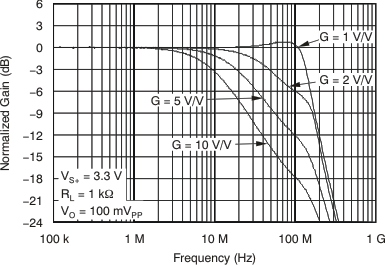Figure 1. Small-Signal Frequency Response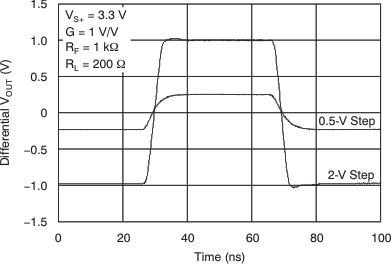Figure 3. Large- and Small-Signal Pulse Response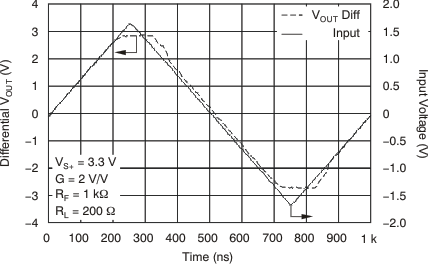Figure 5. Overdrive Recovery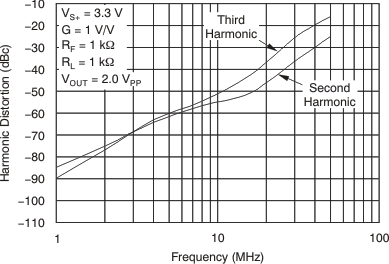Figure 7. Harmonic Distortion vs Frequency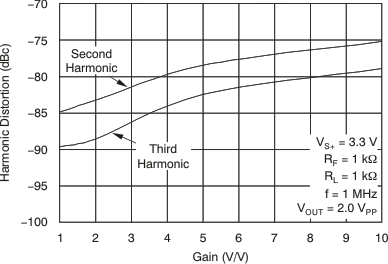Figure 9. Harmonic Distortion vs Gain at 1 MHz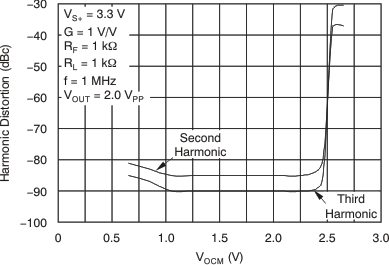Figure 11. Harmonic Distortion vs VOCM at 1 MHz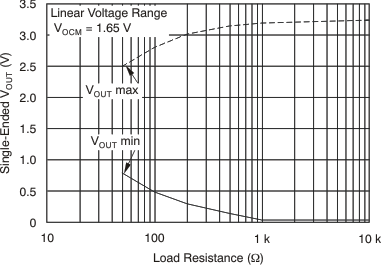Figure 13. Single-Ended Output Voltage Swing vs Load Resistance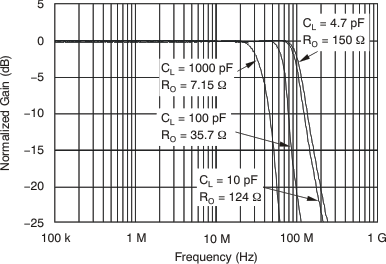Figure 15. Frequency Response vs CLOAD RLOAD = 1 kΩ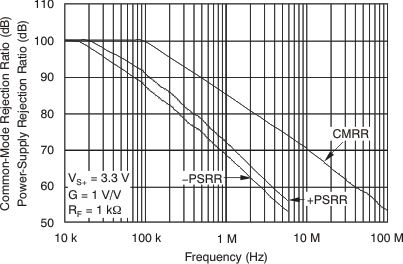Figure 17. Rejection Ratio vs Frequency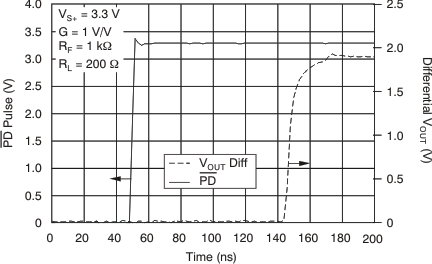Figure 19. Turn-On Time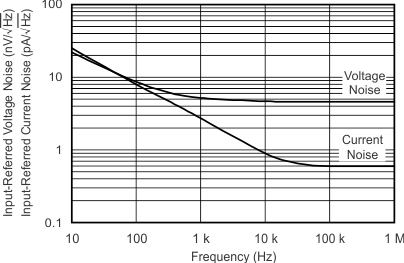Figure 21. Input-Referred Voltage and Current Noise Spectral Density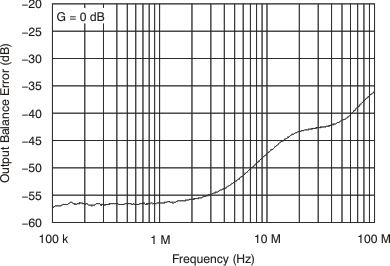Figure 23. Output Balance Error vs Frequency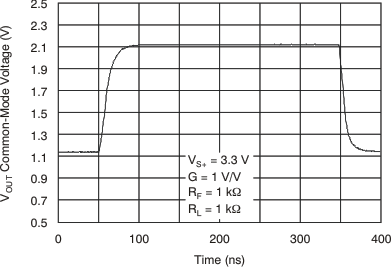Figure 25. VOCM Large-Signal Pulse Response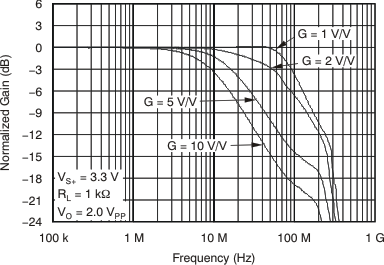Figure 2. Large-Signal Frequency Response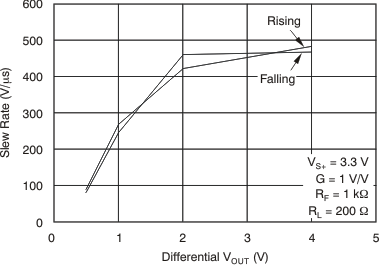Figure 4. Slew Rate vs VOUT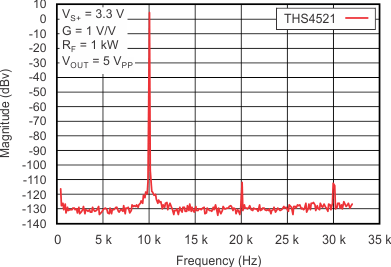Figure 6. 10-kHz Output Spectrum On AP Analyzer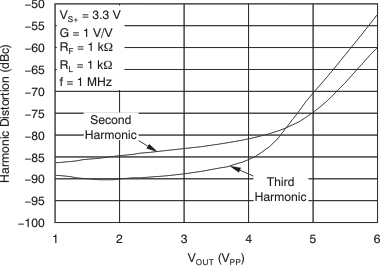Figure 8. Harmonic Distortion vs VOUT at 1 MHz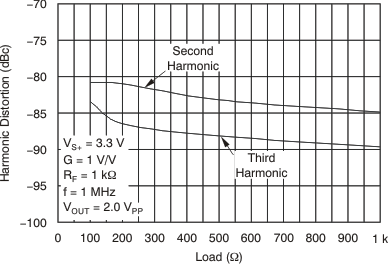Figure 10. Harmonic Distortion vs Load at 1 MHz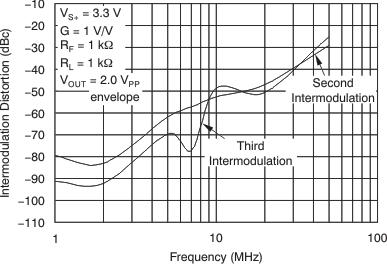Figure 12. Two-Tone Intermodulation Distortion vs Frequency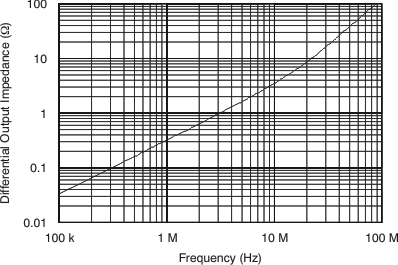Figure 14. Main Amplifier Differential Output Impedance vs Frequency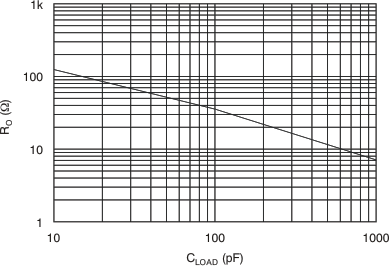Figure 16. RO vs CLOAD RLOAD = 1 kΩFigure 18. THS4522, THS4524 Crosstalk (Differential Measurement)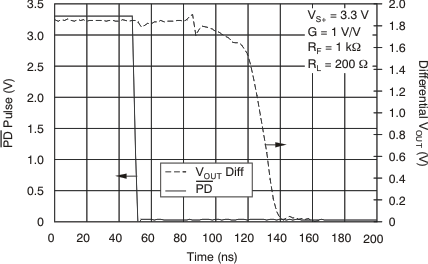Figure 20. Turn-Off Time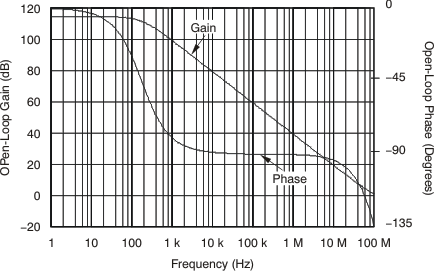Figure 22. Main Amplifier Differential Open-Loop Gain and Phase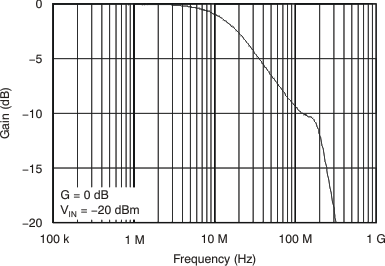Figure 24. VOCM Small-Signal Frequency Response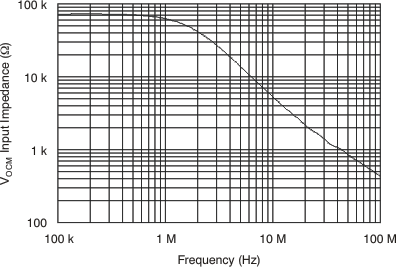Figure 26. VOCM Input Impedance vs Frequency

### 7.9 Typical Characteristics: 5 V

At VS+ = +5 V, VS– = 0 V, VOCM = open, VOUT = 2 VPP (differential), RF = 1 kΩ, RL = 1 kΩ differential, G = 1 V/V, single-ended input, differential output, and input and output referenced to midsupply, unless otherwise noted.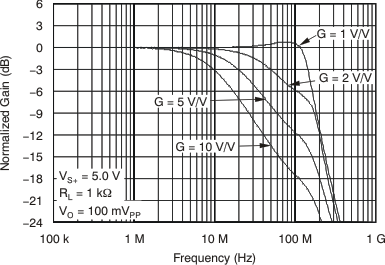Figure 27. Small-Signal Frequency Response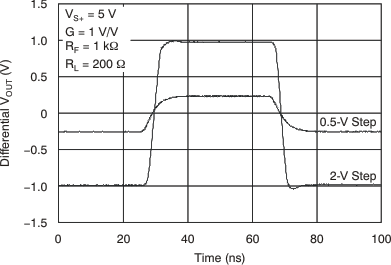Figure 29. Large- and Small-Signal Pulse Response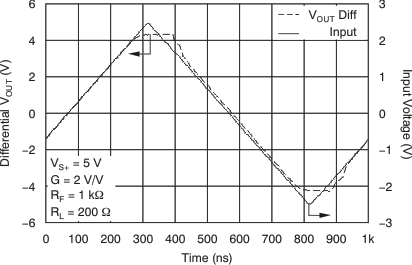Figure 31. Overdrive Recovery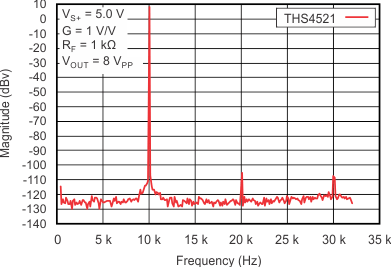Figure 33. 10-kHz Output Spectrum On AP Analyzer at
VOUT = 8 VPP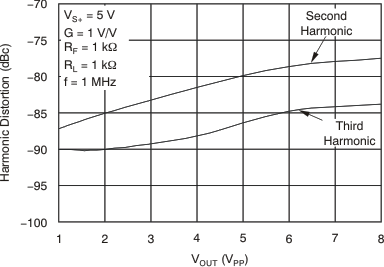Figure 35. Harmonic Distortion vs VOUT at 1 MHz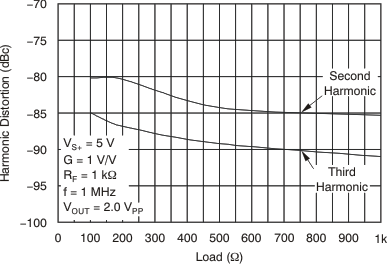Figure 37. Harmonic Distortion vs Load at 1 MHz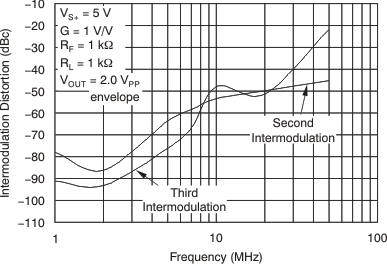Figure 39. Two-Tone Intermodulation Distortion vs Frequency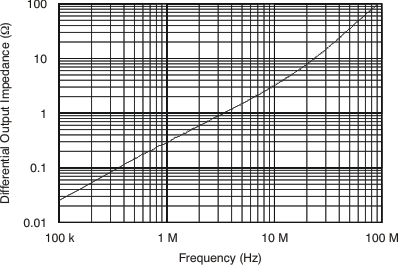Figure 41. Main Amplifier Differential Output Impedance vs Frequency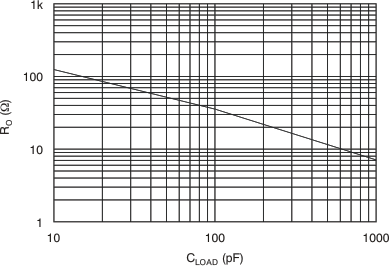Figure 43. RO vs CLOAD RLOAD = 1 kΩ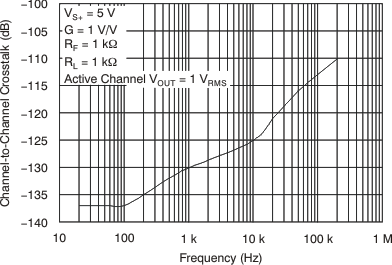Figure 45. THS4522, THS4524 Crosstalk (Measured Differentially)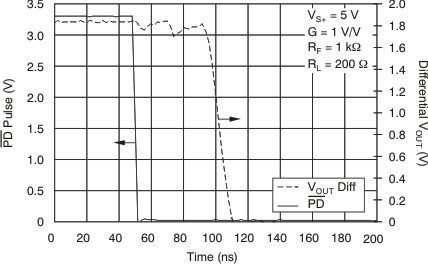Figure 47. Turn-Off Time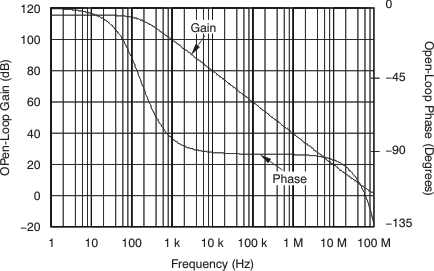Figure 49. Main Amplifier Differential Open-Loop Gain and Phase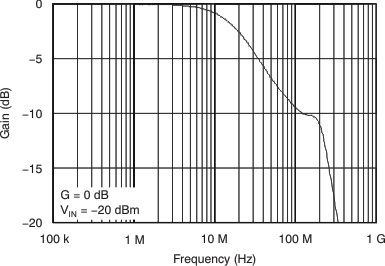Figure 51. VOCM Small-Signal Frequency Response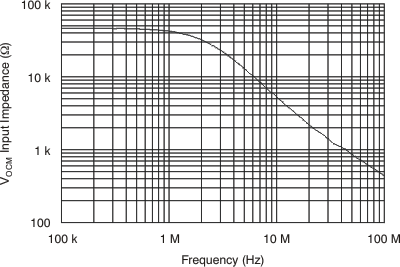Figure 53. VOCM Input Impedance vs Frequency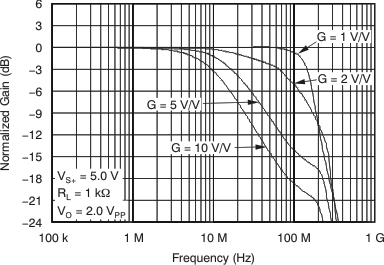Figure 28. Large-Signal Frequency Response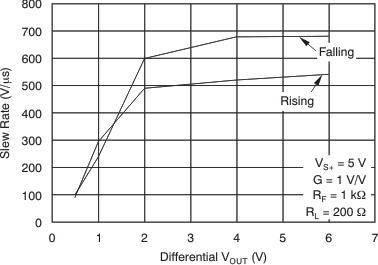Figure 30. Slew Rate vs VOUT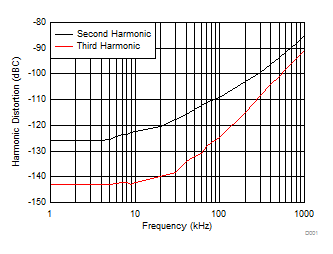Figure 32. Harmonic Distortion vs Frequency Below 1 MHz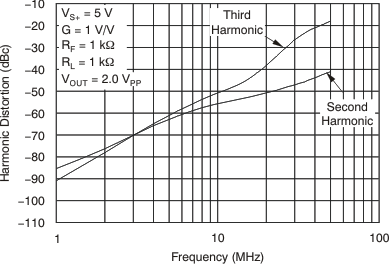Figure 34. Harmonic Distortion vs Frequency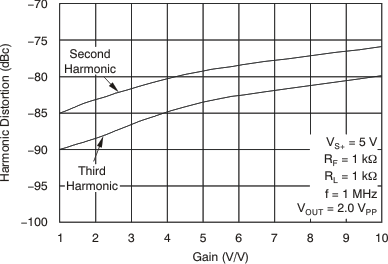Figure 36. Harmonic Distortion vs Gain at 1 MHz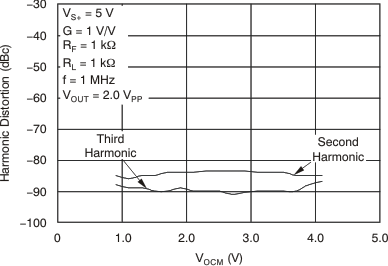Figure 38. Harmonic Distortion vs VOCM at 1 MHz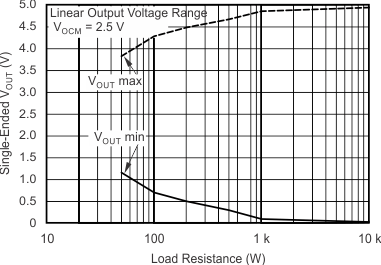Figure 40. Single-Ended Output Voltage Swing vs Differential Load ResistanceFigure 42. Frequency Response vs CLOAD RLOAD = 1 kΩ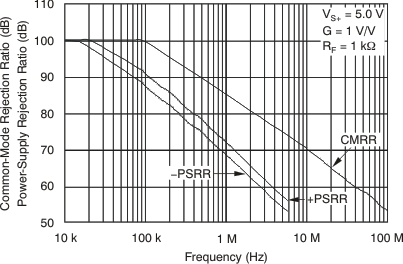Figure 44. Rejection Ratio vs Frequency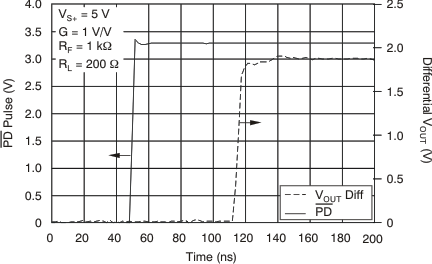Figure 46. Turn-On TimeFigure 48. Input-Referred Voltage and Current Noise Spectral Density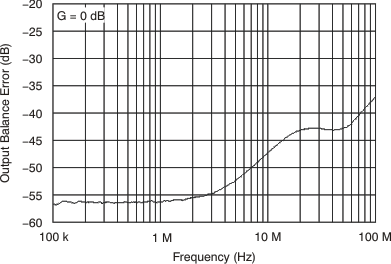Figure 50. Output Balance Error vs Frequency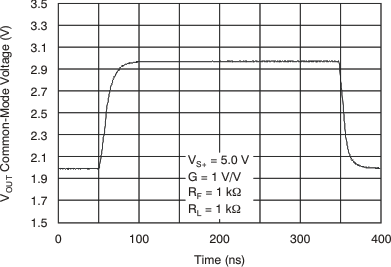Figure 52. VOCM Large-Signal Pulse Response